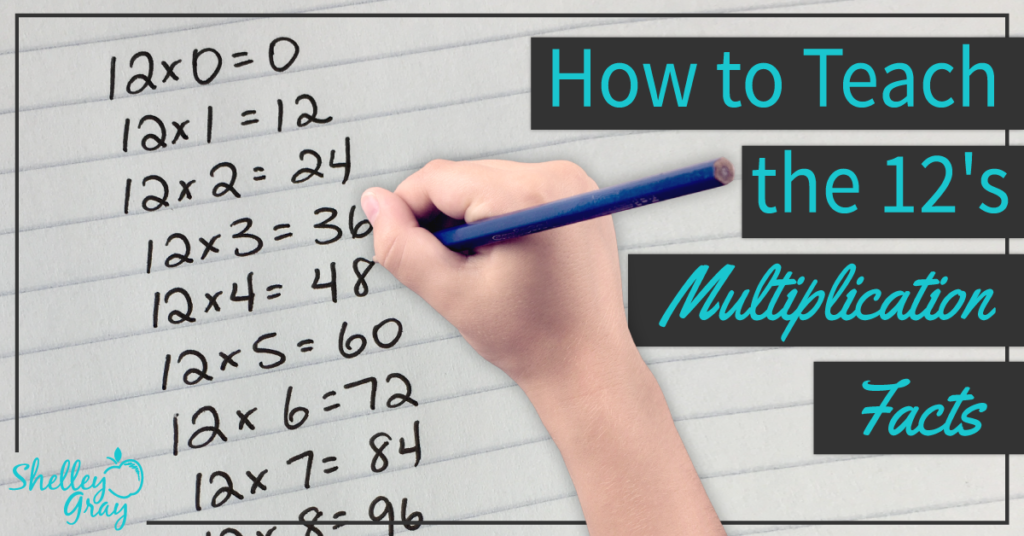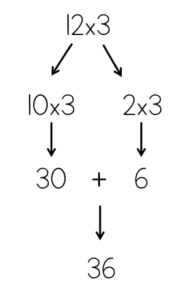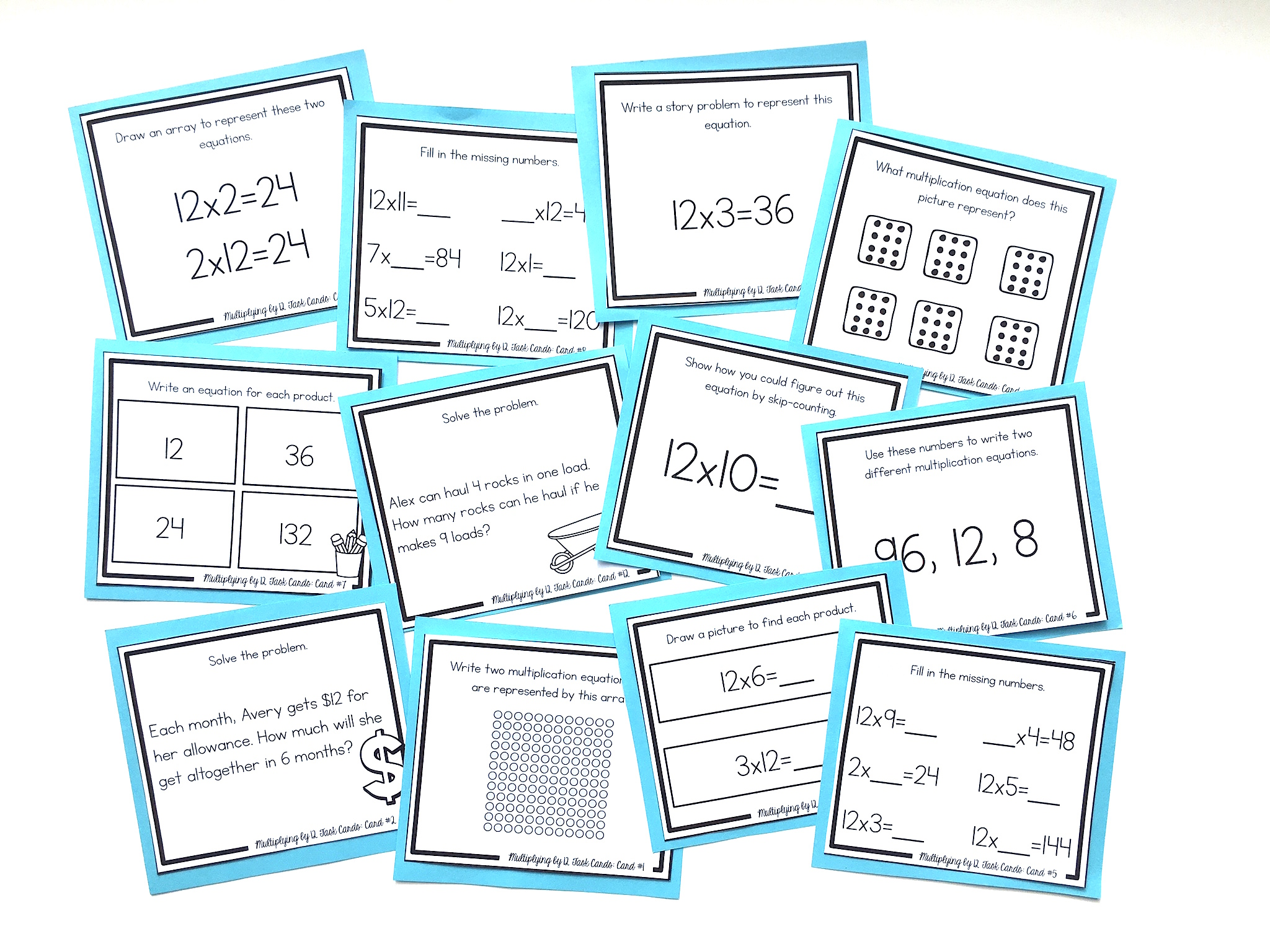# How to Teach the 12 Times Table So It Really Makes SenseYou may not explicitly teach the 12 facts to your students, as many curriculums specify that students are fluent with facts to 10×10. However, solving 12’s facts can be used to teach a very important strategy – breaking apart numbers.

We can use the 12’s to teach our students how to break up numbers and multiply them in groups. In this case, we divide the 12 into a 10 and a 2.

For example, for the equation 12×3, we could divide that 12 into a 10 and a 2, and multiply each part by 3. So 10×3=30, and 2×3=6. Now we can add the 30 and the 6 together to make 36.For the equation 12×5, we could divide that 12 into a 10 and a 2, and multiply each part by 5. So 10×5=50, and 2×5=10. Now we can add the 50 and the 10 together to make 60.It’s also very important to reinforce the commutative property.

This means that the order of factors does not change the product. For example, if students are faced with an equation like 6×12, they should see the 12 as one of the factors, and know that they can use the 12’s strategy to solve this equation.

NEXT STEPS

Reinforce the 12’s multiplication facts with this set of task cards. Students will learn conceptually through problem-solving, using arrays, strategic thinking, finding missing numbers, skip-counting, picture representations, and more:OR FIND THE FULL BUNDLE OF MULTIPLICATION TASK CARDS HERE.